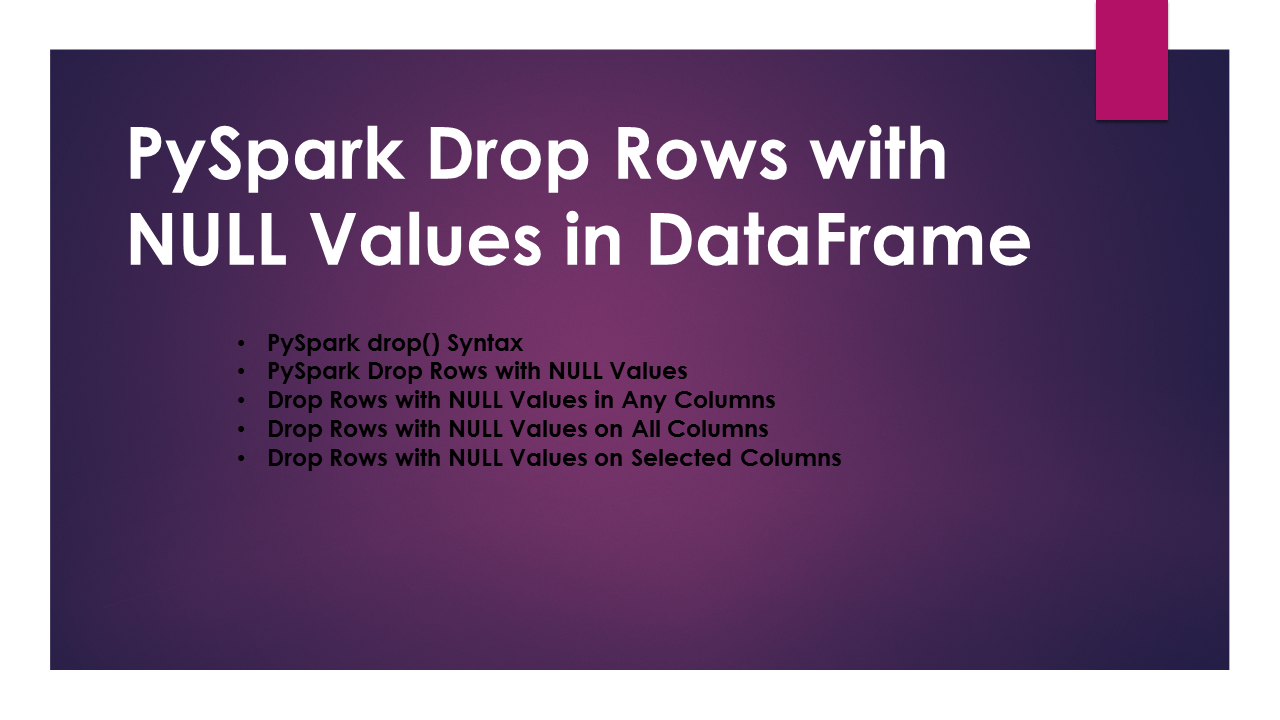Search

# PySpark Drop Rows with NULL or None ValuesIn PySpark, `pyspark.sql.DataFrameNaFunctions` class provides several functions to deal with NULL/None values, among these `drop()` function is used to remove/drop rows with NULL values in DataFrame columns, alternatively, you can also use `df.dropna()`, in this article, you will learn with Python examples.

By using the `drop()` function you can drop all rows with null values in any, all, single, multiple, and selected columns. This function comes in handy when you need to clean the data before processing.

When you read a file into PySpark DataFrame API, any column that has an empty value result in NULL on DataFrame.

In RDBMS SQL, you need to check on every column if the value is null in order to drop however, the PySpark `drop()` function is powerfull as it can checks all columns for null values and drops the rows.

## PySpark drop() Syntax

PySpark `drop()` function can take 3 optional parameters that are used to remove Rows with NULL values on single, any, all, multiple DataFrame columns.

drop() is a transformation function hence it returns a new DataFrame after dropping the rows/records from the current Dataframe.

Syntax:

``````
drop(how='any', thresh=None, subset=None)
``````

All these parameters are optional.

• how – This takes values ‘any’ or ‘all’. By using ‘any’, drop a row if it contains NULLs on any columns. By using ‘all’, drop a row only if all columns have NULL values. Default is ‘any’.
• thresh – This takes int value, Drop rows that have less than thresh hold non-null values. Default is ‘None’.
• subset – Use this to select the columns for NULL values. Default is ‘None.

Alternatively, you can also use DataFrame.dropna() function to drop rows with null values.

## PySpark Drop Rows with NULL Values

DataFrame/Dataset has a variable `na` which is an instance of class `DataFrameNaFunctions` hence, you should be using `na` variable on DataFrame to use drop(). DataFrameNaFunctions class also have method fill() to replace NULL values with empty string on PySpark DataFrame

Before we start, Let’s Read CSV File into DataFrame, when we have no values on certain rows of String and Integer columns, PySpark assigns null values to these empty columns.

The file we are using here is available at GitHub small_zipcode.csv

``````
from pyspark.sql import SparkSession

spark: SparkSession = SparkSession.builder
.master("local")
.appName("SparkByExamples.com")
.getOrCreate()

filePath="resources/small_zipcode.csv"
.csv(filePath)

df.printSchema()
df.show(truncate=False)
``````

This yields the below output. As you see columns type, city and population columns have null values.

``````
+---+-------+--------+-------------------+-----+----------+
|id |zipcode|type    |city               |state|population|
+---+-------+--------+-------------------+-----+----------+
|1  |704    |STANDARD|null               |PR   |30100     |
|2  |704    |null    |PASEO COSTA DEL SUR|PR   |null      |
|3  |709    |null    |BDA SAN LUIS       |PR   |3700      |
|4  |76166  |UNIQUE  |CINGULAR WIRELESS  |TX   |84000     |
|5  |76177  |STANDARD|null               |TX   |null      |
+---+-------+--------+-------------------+-----+----------+
``````

As you see above DataFrame most of the rows have NULL values except record with id=4. Now, let’s see how to drop or remove rows with null values on DataFrame.

## Drop Rows with NULL Values in Any Columns

By default drop() without arguments remove all rows that have null values on any column of DataFrame.

``````
df.na.drop().show(false)
``````

This removes all rows with null values and returns the clean DataFrame with id=4 where it doesn’t have any NULL values.

``````
+---+-------+------+-----------------+-----+----------+
|id |zipcode|type  |city             |state|population|
+---+-------+------+-----------------+-----+----------+
|4  |76166  |UNIQUE|CINGULAR WIRELESS|TX   |84000     |
+---+-------+------+-----------------+-----+----------+
``````

Alternatively you can also get same result with `na.drop("any")`.

``````
df.na.drop("any").show(false)
``````

## Drop Rows with NULL Values on All Columns

Below example drops all rows that has NULL values on all columns. Our DataFrame doesn’t have null values on all rows hence below examples returns all rows.

``````
df.na.drop("all").show(false)
``````

## Drop Rows with NULL Values on Selected Columns

In order to remove Rows with NULL values on selected columns of PySpark DataFrame, use drop(columns:Seq[String]) or drop(columns:Array[String]). To these functions pass the names of the columns you wanted to check for NULL values to delete rows.

``````
df.na.drop(subset=["population","type"])
.show(truncate=False)
``````

The above example remove rows that have NULL values on `population` and `type` selected columns.

``````
+---+-------+--------+-----------------+-----+----------+
|id |zipcode|type    |city             |state|population|
+---+-------+--------+-----------------+-----+----------+
|1  |704    |STANDARD|null             |PR   |30100     |
|4  |76166  |UNIQUE  |CINGULAR WIRELESS|TX   |84000     |
+---+-------+--------+-----------------+-----+----------+
``````

## Using dropna() of DataFrame

Below is a PySpark example of using `dropna()` function of DataFrame to drop rows with NULL values.

``````
df.dropna().show(truncate=False)
``````

## Complete Example of Drop rows with NULL Values

Below is a complete Spark example of using `drop()` and `dropna()` for reference.

``````
from pyspark.sql import SparkSession

spark: SparkSession = SparkSession.builder
.master("local")
.appName("SparkByExamples.com")
.getOrCreate()

filePath="resources/small_zipcode.csv"
.csv(filePath)

df.printSchema()
df.show(truncate=False)

df.na.drop().show(truncate=False)

df.na.drop(how="any").show(truncate=False)

df.na.drop(subset=["population","type"])
.show(truncate=False)

df.dropna().show(truncate=False)
``````

This complete code is available at GitHub project.

## Conclusion

In this PySpark article, you have learned how to delete/remove/drop rows with NULL values in any, all, sing, multiple columns in Dataframe using drop() function of `DataFrameNaFunctions` and `dropna()` of DataFrame with Python example.

Happy Learning !!

#### Barbara Cartland

You should write because you love the shape of Flow and sentences and the creation of different words on a page.

####Subscribe